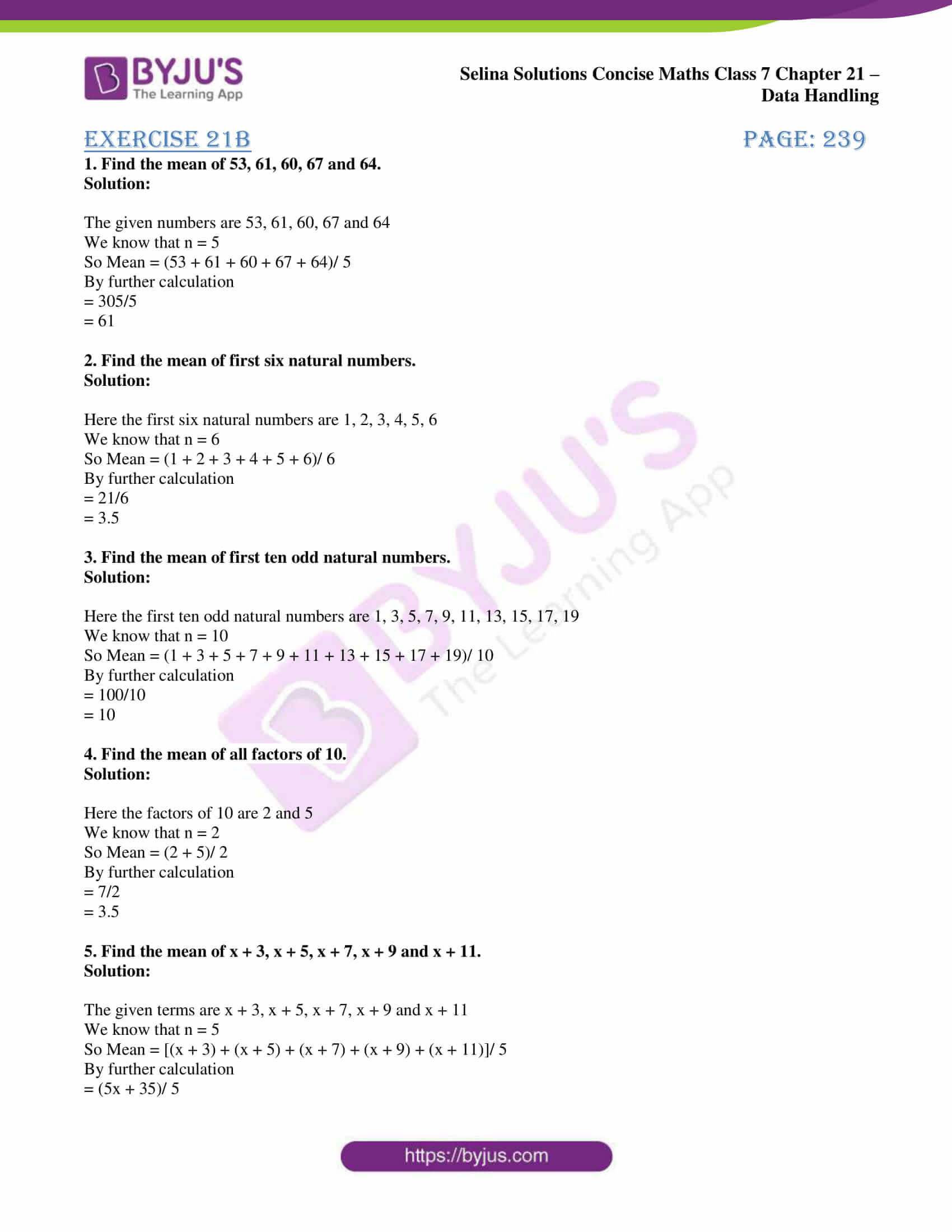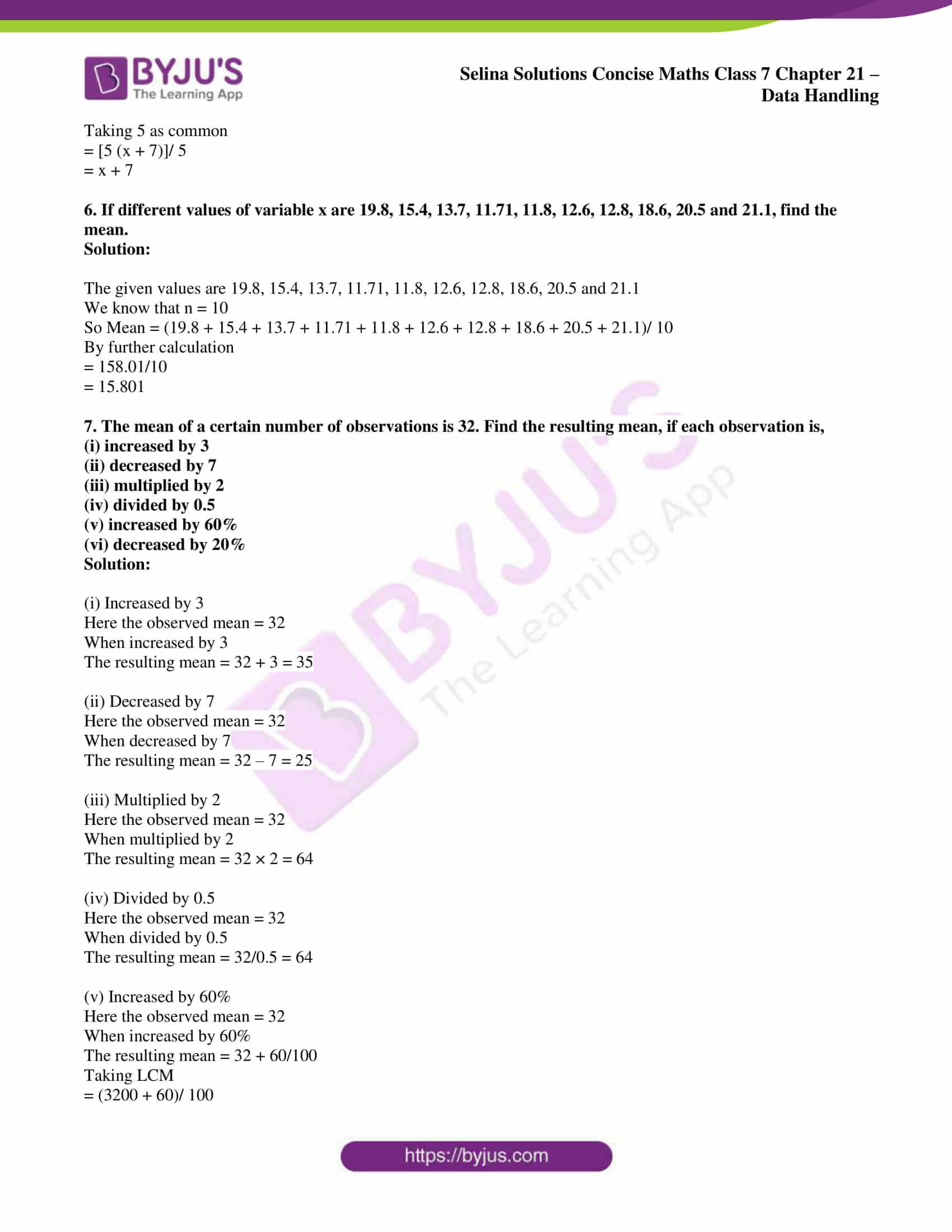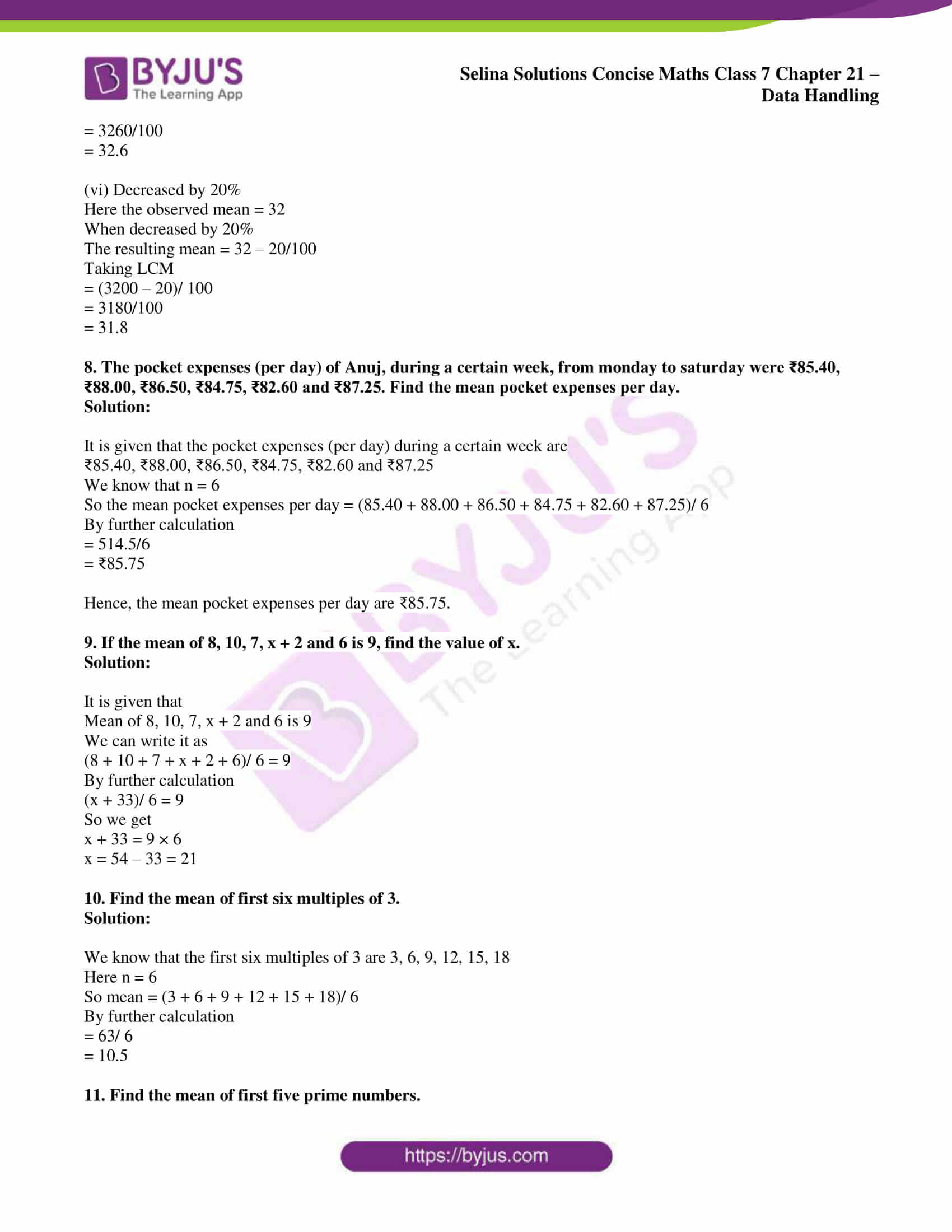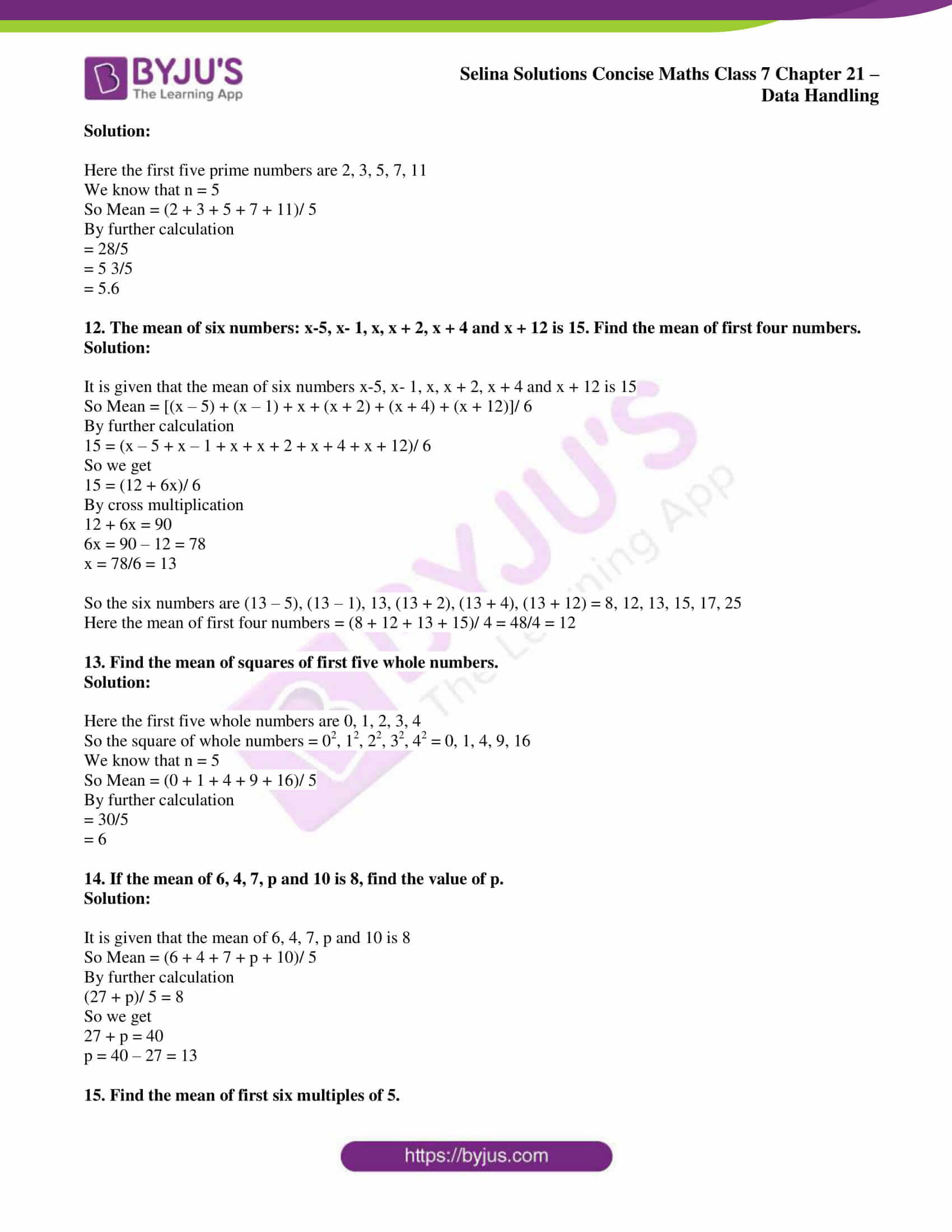# Selina Solutions Concise Maths Class 7 Chapter 21: Data Handling Exercise 21B

Selina Solutions Concise Maths Class 7 Chapter 21 Data Handling Exercise 21B provides students a clear idea about the concepts, which are important for the exam. The solutions created by the subject matter experts are accurate, based on the latest syllabus of ICSE board. The method of finding the mean, median, mode and bar graph are the major concepts covered here. Important formulas and shortcut methods are explained in simple language to boost problem solving abilities among students. To ace the annual exam, students can avail Selina Solutions Concise Maths Class 7 Chapter 21 Data Handling Exercise 21B PDF, from the links which are available here.

## Selina Solutions Concise Maths Class 7 Chapter 21: Data Handling Exercise 21B Download PDF### Access Selina Solutions Concise Maths Class 7 Chapter 21: Data Handling Exercise 21B

Exercise 21B page: 239

1. Find the mean of 53, 61, 60, 67 and 64.

Solution:

The given numbers are 53, 61, 60, 67 and 64

We know that n = 5

So Mean = (53 + 61 + 60 + 67 + 64)/ 5

By further calculation

= 305/5

= 61

2. Find the mean of first six natural numbers.

Solution:

Here the first six natural numbers are 1, 2, 3, 4, 5, 6

We know that n = 6

So Mean = (1 + 2 + 3 + 4 + 5 + 6)/ 6

By further calculation

= 21/6

= 3.5

3. Find the mean of first ten odd natural numbers.

Solution:

Here the first ten odd natural numbers are 1, 3, 5, 7, 9, 11, 13, 15, 17, 19

We know that n = 10

So Mean = (1 + 3 + 5 + 7 + 9 + 11 + 13 + 15 + 17 + 19)/ 10

By further calculation

= 100/10

= 10

4. Find the mean of all factors of 10.

Solution:

Here the factors of 10 are 2 and 5

We know that n = 2

So Mean = (2 + 5)/ 2

By further calculation

= 7/2

= 3.5

5. Find the mean of x + 3, x + 5, x + 7, x + 9 and x + 11.

Solution:

The given terms are x + 3, x + 5, x + 7, x + 9 and x + 11

We know that n = 5

So Mean = [(x + 3) + (x + 5) + (x + 7) + (x + 9) + (x + 11)]/ 5

By further calculation

= (5x + 35)/ 5

Taking 5 as common

= [5 (x + 7)]/ 5

= x + 7

6. If different values of variable x are 19.8, 15.4, 13.7, 11.71, 11.8, 12.6, 12.8, 18.6, 20.5 and 21.1, find the mean.

Solution:

The given values are 19.8, 15.4, 13.7, 11.71, 11.8, 12.6, 12.8, 18.6, 20.5 and 21.1

We know that n = 10

So Mean = (19.8 + 15.4 + 13.7 + 11.71 + 11.8 + 12.6 + 12.8 + 18.6 + 20.5 + 21.1)/ 10

By further calculation

= 158.01/10

= 15.801

7. The mean of a certain number of observations is 32. Find the resulting mean, if each observation is,

(i) increased by 3

(ii) decreased by 7

(iii) multiplied by 2

(iv) divided by 0.5

(v) increased by 60%

(vi) decreased by 20%

Solution:

(i) Increased by 3
Here the observed mean = 32

When increased by 3

The resulting mean = 32 + 3 = 35

(ii) Decreased by 7
Here the observed mean = 32

When decreased by 7

The resulting mean = 32 – 7 = 25

(iii) Multiplied by 2
Here the observed mean = 32

When multiplied by 2

The resulting mean = 32 × 2 = 64

(iv) Divided by 0.5
Here the observed mean = 32

When divided by 0.5

The resulting mean = 32/0.5 = 64

(v) Increased by 60%
Here the observed mean = 32

When increased by 60%

The resulting mean = 32 + 60/100

Taking LCM

= (3200 + 60)/ 100

= 3260/100

= 32.6

(vi) Decreased by 20%

Here the observed mean = 32

When decreased by 20%

The resulting mean = 32 – 20/100

Taking LCM

= (3200 – 20)/ 100

= 3180/100

= 31.8

8. The pocket expenses (per day) of Anuj, during a certain week, from monday to saturday were ₹85.40, ₹88.00, ₹86.50, ₹84.75, ₹82.60 and ₹87.25. Find the mean pocket expenses per day.

Solution:

It is given that the pocket expenses (per day) during a certain week are

₹85.40, ₹88.00, ₹86.50, ₹84.75, ₹82.60 and ₹87.25

We know that n = 6

So the mean pocket expenses per day = (85.40 + 88.00 + 86.50 + 84.75 + 82.60 + 87.25)/ 6

By further calculation

= 514.5/6

= ₹85.75

Hence, the mean pocket expenses per day are ₹85.75.

9. If the mean of 8, 10, 7, x + 2 and 6 is 9, find the value of x.

Solution:

It is given that

Mean of 8, 10, 7, x + 2 and 6 is 9

We can write it as

(8 + 10 + 7 + x + 2 + 6)/ 6 = 9

By further calculation

(x + 33)/ 6 = 9

So we get

x + 33 = 9 × 6

x = 54 – 33 = 21

10. Find the mean of first six multiples of 3.

Solution:

We know that the first six multiples of 3 are 3, 6, 9, 12, 15, 18

Here n = 6

So mean = (3 + 6 + 9 + 12 + 15 + 18)/ 6

By further calculation

= 63/ 6

= 10.5

11. Find the mean of first five prime numbers.

Solution:

Here the first five prime numbers are 2, 3, 5, 7, 11

We know that n = 5

So Mean = (2 + 3 + 5 + 7 + 11)/ 5

By further calculation

= 28/5

= 5 3/5

= 5.6

12. The mean of six numbers: x-5, x- 1, x, x + 2, x + 4 and x + 12 is 15. Find the mean of first four numbers.

Solution:

It is given that the mean of six numbers x-5, x- 1, x, x + 2, x + 4 and x + 12 is 15

So Mean = [(x – 5) + (x – 1) + x + (x + 2) + (x + 4) + (x + 12)]/ 6

By further calculation

15 = (x – 5 + x – 1 + x + x + 2 + x + 4 + x + 12)/ 6

So we get

15 = (12 + 6x)/ 6

By cross multiplication

12 + 6x = 90

6x = 90 – 12 = 78

x = 78/6 = 13

So the six numbers are (13 – 5), (13 – 1), 13, (13 + 2), (13 + 4), (13 + 12) = 8, 12, 13, 15, 17, 25

Here the mean of first four numbers = (8 + 12 + 13 + 15)/ 4 = 48/4 = 12

13. Find the mean of squares of first five whole numbers.

Solution:

Here the first five whole numbers are 0, 1, 2, 3, 4

So the square of whole numbers = 02, 12, 22, 32, 42 = 0, 1, 4, 9, 16

We know that n = 5

So Mean = (0 + 1 + 4 + 9 + 16)/ 5

By further calculation

= 30/5

= 6

14. If the mean of 6, 4, 7, p and 10 is 8, find the value of p.

Solution:

It is given that the mean of 6, 4, 7, p and 10 is 8

So Mean = (6 + 4 + 7 + p + 10)/ 5

By further calculation

(27 + p)/ 5 = 8

So we get

27 + p = 40

p = 40 – 27 = 13

15. Find the mean of first six multiples of 5.

Solution:

Here the first six multiples of 5 are 5, 10, 15, 20, 25 and 30

We know that n = 6

So Mean = (5 + 10 + 15 + 20 + 25 + 30)/ 6

By further calculation

= 105/6

= 17.5